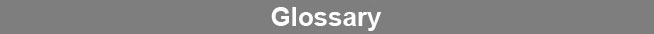Select Page### Logistic Regressions

A five parameter logistic curve describes a nonlinear curve such as that found with most immunoassay and bioassay dilution curves. Logistic curves are defined with five coefficients: Coefficients a and d are the high and low asymptotes. Coefficient b directs the shape of the curve. Coefficient c is the inflection point of the curve. Coefficient g is the asymmetry parameter, and with coefficient b directs the shape of the curve. When the curve is symmetrical and the point of inflection is at the midpoint of the curve, coefficient g equals 1 and the equation becomes a four parameter logistic curve.www.brendan.com